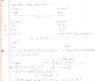# Problem with Classic where do they meet Problem

• derekfuselier

#### derekfuselier

Problem with Classic "where do they meet" Problem

I have always had problems with these types of concepts because no one has ever showed me exactly what is required to solve the problem. Anyways, here it is:

In an historical movie, two knights on horseback start from rest 99.8 m apart and ride directly toward each other to do battle. Sir George's acceleration has a magnitude of 0.207 m/s^2, while Sir Alfred's has a magnitude of 0.229 m/s^2. Relative to Sir George's starting point, where do the knights collide?

I was instructed to, using the kinematic equation Xf = Xi + (Vi)(t) + (1/2)(a)(t)^2, find t^2 and sub into the other equation, but doing that leaves me with a variable, x, on each side of the equation. Any help would be extremely appreciated, thanks!

How did you set up your equations? Take George to start at point x=0, and Arthur to start at point x=99.8, and use the equation you have written (one for each horse). Now, when the knights meet, what can you say about the displacements of the two knights? Can you calculate t from this? If so, then you should be able to find x.

Show your working, and we will be able to help!

Last edited:
Can you show how you substituted?

What values were you using for? e.g.
Knight1 - Xi, Vi, a
Knight2 - Xi, Vi, a

Sorry guys, let me draw it up and scan then I'll show my work. Thanks so much!

Ok, my work is attached.

#### Attachments

•horse.JPG
26.9 KB · Views: 416
How did you set up your equations? Take George to start at point x=0, and Arthur to start at point x=99.8, and use the equation you have written (one for each horse). Now, when the knights meet, what can you say about the displacements of the two knights? Can you calculate t from this? If so, then you should be able to find x.

Show your working, and we will be able to help!

Well George would be displaced by x and Albert by 99.8 - x, right? If not, blame the Louisiana schools ;)

Albert will be displaced from his starting point by 99.8-x, but the question asks for the displacement of him relative to george's starting point

So, you need to set up a coordinate system with origin on george's starting point. Thus, relative to this coordinate system, what are the accelerations of each knight?

(I can't see your attachment yet; it needs to be approved, but I'll try and hurry the process up!)

Albert will be displaced from his starting point by 99.8-x, but the question asks for the displacement of him relative to george's starting point

So, you need to set up a coordinate system with origin on george's starting point. Thus, relative to this coordinate system, what are the accelerations of each knight?

(I can't see your attachment yet; it needs to be approved, but I'll try and hurry the process up!)

I understand that they are asking for distance relative to George's position. However, I'm not quite sure what you mean by this coordinate system.

Ok, I've seen your work now. (I like your pictures of horses!) I've not checked your arithmetic, but youv'e made a mistake in calculating t.

Note that, you have two equations for xf, so set them equal to each other and you will get an expression $$\frac{1}{2}0.207t^2=99.8-\frac{0.229}{2}t^2$$ (1)

Solve this for t, and then sub into one of the equations to obtain xf

(Sorry, the first time I glanced through and didn't pick this up. I only noticed when I calculated xf from your last equation and it turned out to be >99.8. So, if you read my first response, I apologise as my commenets were not correct!)

edit:
I understand that they are asking for distance relative to George's position. However, I'm not quite sure what you mean by this coordinate system.

Ignore that; I was just hinting at the fact that one acceleration is negative, but after looking at your work, I can see that you know this!

Last edited:
Maybe I just can't see how to solve for t because I've been working problems for too long or I could just be an idiot.

Maybe I just can't see how to solve for t because I've been working problems for too long or I could just be an idiot.

Id go for the former reason! In my equation (1) in my last post, simplify it by putting all the terms involving t2 onto one side. Then you'll have an expression At2=99.8, where A is a number. Divide both sides by A, take the square root, and you will obtain the value of t.

Then, sub this into one of the equations for xf in your work, and you will obtain xf

Id go for the former reason! In my equation (1) in my last post, simplify it by putting all the terms involving t2 onto one side. Then you'll have an expression At2=99.8, where A is a number. Divide both sides by A, take the square root, and you will obtain the value of t.

Then, sub this into one of the equations for xf in your work, and you will obtain xf

Lol, thanks! But I still don't see how to put them on the same sideTo me, algebraically, to get them on the same side on must divide by t^2 which would cancel out the other t^2. I'm feeling especially dense right now.

Lol, oh my. Subtract, factor out, etc..what's wrong with me? Thanks!

Last edited: